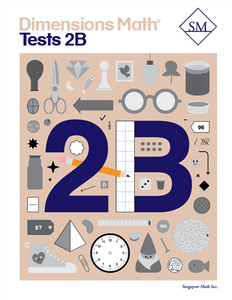# Dimensions Math Tests 2B

Vendor
Singapore Math Inc.
Regular price
\$13.70
Sale price
\$13.70
Quantity must be 1 or more

The Dimensions Math® PK-5 series is a new curriculum designed by Singapore Math Inc. to better serve U.S. teachers and students. Aspects of Singapore math curriculum have been updated for clarity and relevance, while preserving the solid foundation that makes it unique. The series follows the principles outlined in the Singapore Mathematics Framework and uses the Concrete > Pictorial > Abstract approach. The curriculum also incorporates Singapore mental math strategies and model drawing.

In the Dimensions Math program, concepts are introduced and explained in a new format with vibrant imagery. The implementation of this curriculum suits today’s needs, while the progression and scope that define Singapore math remain intact.

The Dimensions Math Tests books offer a series of assessment to help teachers and parents systematically evaluate student progress.

Samples of Dimensions Math Tests 2B

Chapter 8: Mental Calculation
Test A
Test B

Chapter 9: Multiplication and Division of 3 and 4
Test A
Test B

Chapter 10: Money
Test A
Test B

Chapter 11: Fractions
Test A
Test B

Continual Assessment 3
Test A
Test B

Chapter 12: Time
Test A
Test B

Chapter 13: Capacity
Test A
Test B

Chapter 14: Graphs
Test A
Test B

Chapter 15: Shapes
Test A
Test B

Year-End Assessment
Test A
Test B